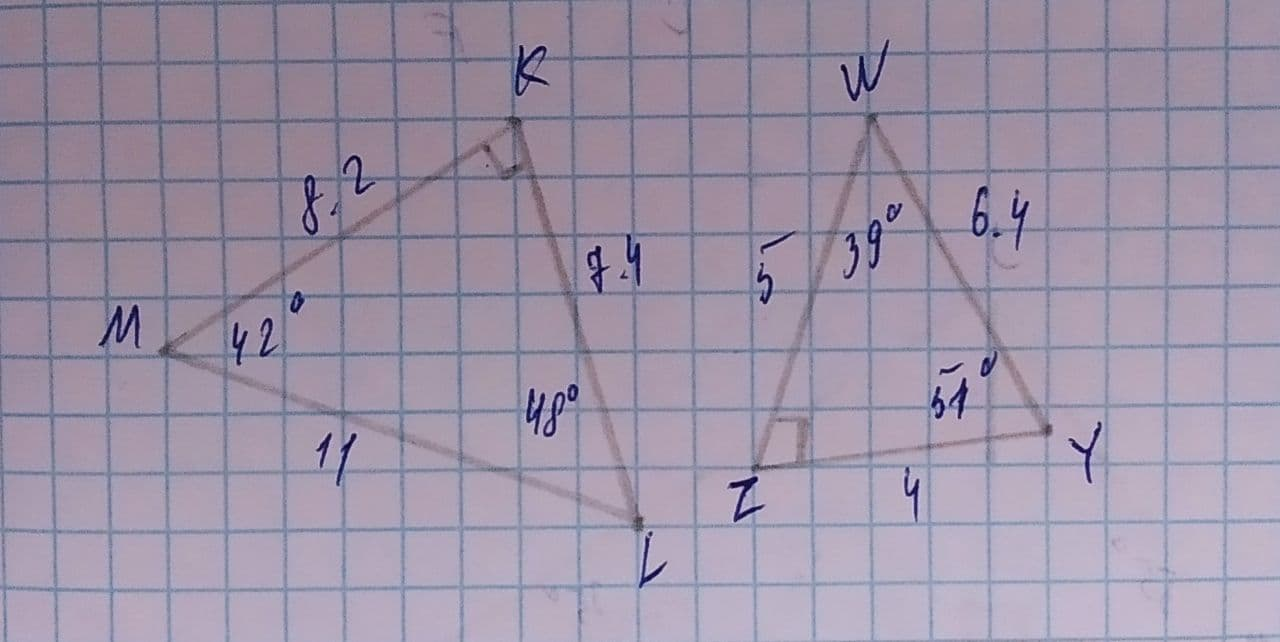# Determine whatever each pair if figures is similar. If so, write the similarity statement and scale factor. If not, explain your reasoning.nagasenaz 2021-07-31 Answered
Determine whatever each pair if figures is similar. If so, write the similarity statement and scale factor. If not, explain your reasoning.You can still ask an expert for help

• Questions are typically answered in as fast as 30 minutes

Solve your problem for the price of one coffee

• Math expert for every subject
• Pay only if we can solve itwornoutwomanC
Step 1
Given,
$\mathrm{△}KLM$
with angles
and sides KL=7.4, ML=11 and KM=8.2
$\mathrm{△}WYZ$
with angles
and sides WY=6.4, YZ=4 and WZ=5
We have to find the similarity between the and scale factor
Step 2
We know
In similar triangles , all the three angles are same
We have
$\mathrm{△}KLM$ with angles
and
$\mathrm{△}WYZ$ with angles
So,
All the angles are not same .
Then,
are not similar.